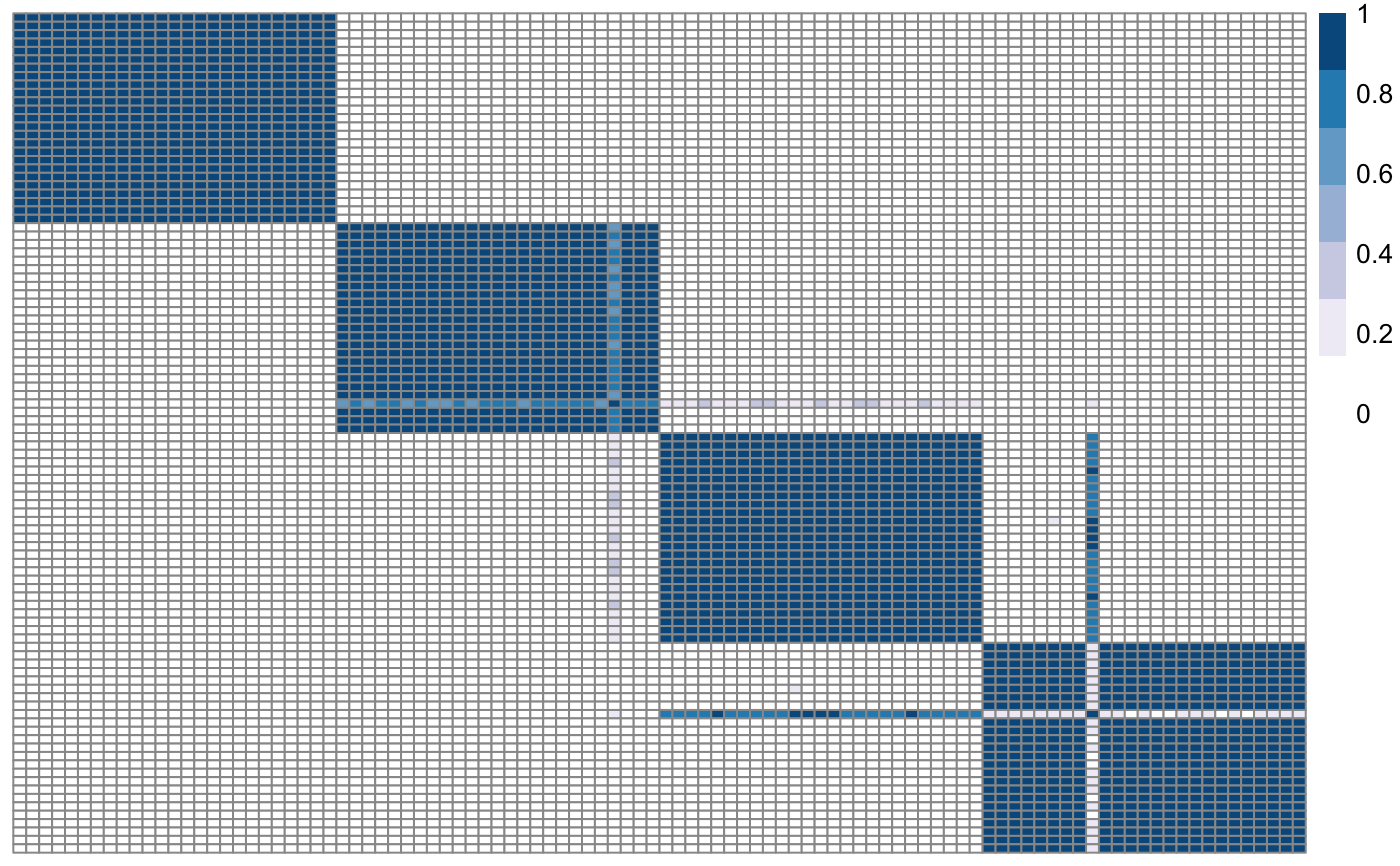Plot similarity matrix with pheatmap

plotSimilarityMatrix(
X,
y = NULL,
clusLabels = NULL,
colX = NULL,
colY = NULL,
myLegend = NULL,
fileName = "posteriorSimilarityMatrix",
savePNG = FALSE,
semiSupervised = FALSE,
showObsNames = FALSE,
clr = FALSE,
clc = FALSE,
plotWidth = 500,
plotHeight = 450
)

## Arguments

X Similarity matrix. Vector Cluster labels Colours for the matrix Colours for the response Vector of strings with the names of the variables If savePNG is TRUE, this is the string containing the name of the output file. Can be used to specify the folder path too. Default is "posteriorSimilarityMatrix". The extension ".png" is automatically added to this string. Boolean: if TRUE, the plot is saved as a png file. Default is FALSE. Boolean flag: if TRUE, the response is plotted next to the matrix. Boolean. If TRUE, observation names are shown in the plot. Default is FALSE. Boolean. If TRUE, rows are ordered by hierarchical clustering. Default is FALSE. Boolean. If TRUE, columns are ordered by hierarchical clustering. Default is FALSE. Plot width. Default is 500. Plot height. Default is 450.

## Value

No return value. This function plots the similarity matrix either to screen or to a png file.

## Examples

# Load one dataset with 100 observations, 2 variables, 4 clusters
package = "klic"), row.names = 1))
plotSimilarityMatrix(cm)plotSimilarityMatrix(cm, y = cluster_labels)